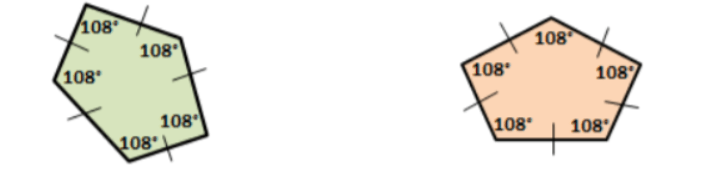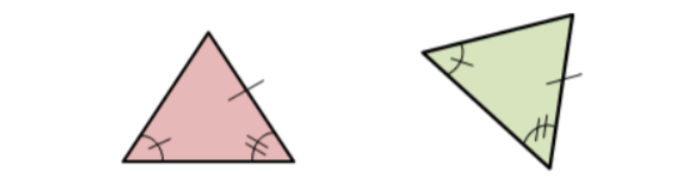# Triangle Congruence by ASA and AAS

After students familiarize themselves with congruent figures, they move on to triangle congruence by ASA and AAS. More specifically, they learn how to prove triangles are congruent using ASA and AAS.

If you’re teaching this topic and wondering how to make these lessons accessible and exciting for your students – we’ve got you covered! Use the teaching strategies that we share in this article and make the class atmosphere as inviting as it gets!## How to Teach Triangle Congruence by ASA and AAS

### Review Congruent Figures

You can start your lesson on triangle congruence by ASA and AAS by providing a brief review of what congruent figures are. Remind students that we define congruent figures as figures that have the same shape and the same size.

Also, add that the corresponding angles of two congruent figures are equal and the corresponding sides are equal. Draw an example on the whiteboard of two figures that are congruent, such as the figures below:Point out that these two figures are congruent because we can easily observe that they have the same shape (they are both pentagons) and they also have the same size.

You can also remind students of the difference between congruent and similar figures. While congruent figures have the same shape and size, similar figures have the same shape, but different sizes.

### What Is Triangle Congruence by ASA and AAS?

Explain to students that if two angles and their included side of one triangle are congruent to the two angles and their included side of another triangle, then the two triangles are said to be congruent.

We call this triangle congruence by ASA or angle-side-angle. Present an example of two triangles that are congruent by the ASA postulate on the whiteboard, such as the following two triangles:Ask students to reflect. Why are these two triangles congruent by the ASA theorem? We notice that the congruent side is in between the two congruent angles, that is, there is triangle congruence by angle-side-angle or ASA.

Now you can move on to explain triangle congruence by AAS. Point out that if two angles and their non-included side of one triangle are congruent to the corresponding two angles and their non-included side of another triangle, the two triangles are congruent.

We call this triangle congruence by AAS or angle-angle-side. Present an example of two triangles that are congruent by the AAS postulate on the whiteboard, such as the following two triangles:Ask students to reflect again. Why are these two triangles congruent by the AAS theorem? We notice that we have two congruent angles, and the side is outside or not included between these two angles. Therefore, there is triangle congruence by angle-angle-side or AAS.

### Launch a Video Lesson!

If you have the technical means in your classroom, enrich your lesson on triangle congruence by ASA and AAS by including multimedia material, such as videos. For instance, use this video by Khan Academy to introduce triangle congruence by ASA and AAS.

To illustrate the difference between triangle congruence by ASA and AAS, play this video. It contains simple explanations of the two, as well as their differences. It also includes short exercises to decide which postulate to use for a set of triangles.

## Activities to Practice Angle Congruence by ASA and AAS### Pair Work

This activity will help students practice identifying and proving whether two triangles are congruent using ASA and AAS. To implement this activity in your classroom, print out this Assignment Worksheet (Members Only).

Pair students up and hand out the copies (one copy per child). Explain that the worksheet contains different math problems, where students are asked to identify whether the given pair

of triangles are congruent or not, as well as state the postulate by which they are congruent.

Students work individually to complete their worksheet. After they’re done, they switch the worksheets with the other person in their pair. They review each other’s work and provide feedback.

### Congruent Triangles Game

This is a simple online game that will help students reinforce their skills at identifying and proving if two triangles are congruent. The game is a bit advanced, so make sure you use it once you’ve covered the SSS (side-side-side), SAS (side-angle-side), ASA, and AAS postulates.

To use this activity in your classroom, make sure you have enough technical devices (one device per student). Pair students up and provide instructions. Explain that students are presented with images of triangles that may or may not be congruent.

Students are offered multiple answers of postulates regarding triangle congruence of the given image, and they have to pick one. In the end, they check their score and compare it with the other student in their pair. The student with the highest score wins the game.

### Congruent Triangles Sorting Activity

This is a sorting activity that will help students practice identifying whether given sets of triangles are congruent either by ASA or AAS. To implement this activity in your classroom, you’ll need plenty of boxes, scissors, paper and an answer sheet.

Draw diverse sets of triangles that are congruent by ASA or AAS. Cut the different sets and create a pile. You can also laminate the sets and re-use them next year. Then, take two boxes and write ASA on one box and AAS on the other one.

Repeat this procedure until you have enough sets and boxes for 5 or 6 groups (depending on the size of your class). Divide students into groups of 3 and hand out two boxes and a pile of triangle sets. Provide instructions for the activity.

Identify one checker in each group and give them the answer sheet. Explain that the other two students in each group work together to sort the sets of triangles either in the ASA box or the AAS box, depending on whether they think the triangles are congruent by ASA or AAS.

Provide a few minutes for this. Once the time is up, the checker checks how many triangle sets the two students managed to correctly sort out. For each correctly sorted set, they earn one point. The group with the highest score is declared the winner.

## Before You Leave…

If you liked our strategies on teaching triangle congruence by ASA and AAS, and you’re looking for more math resources for kids of all ages, sign up for our emails to receive loads of free resources, including worksheets, guided lesson plans and notes, activities, and much more!

Feel free to also head over to our blog, where you’ll find plenty of free and awesome resources! And if you’d like to become a member, join us in the Math Teacher Coach community!